Next: Wave Propagation in Inhomogeneous Up: Wave Propagation in Uniform Previous: Signal Arrival

# Exercises

1. A general electromagnetic wave pulse propagating in the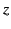-direction at velocity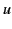is written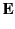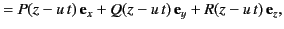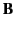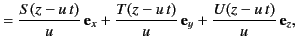where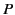,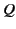,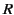,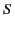,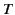, and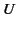are arbitrary functions. In order to exclude electrostatic and magnetostatic fields, these functions are subject to the constraint that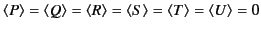, where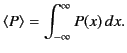Suppose that the pulse propagates through a uniform dielectric medium of dielectric constant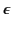. Demonstrate from Maxwell's equation that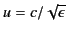,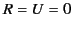,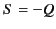, and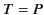. Incidentally, this result implies that a general wave pulse is characterized by two arbitrary functions, corresponding to the two possible independent polarizations of the pulse.

2. Show that the mean energy flux due to an electromagnetic wave of angular frequency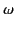propagating though a good conductor of conductivity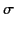can be written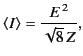where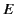is the peak electric field-strength, and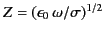.

3. Consider an electromagnetic wave propagating in the positive-direction through a good conductor of conductivity. Suppose that the wave electric field is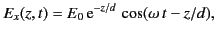where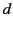is the skin-depth. Demonstrate that the mean electromagnetic energy flux across the plane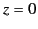matches the mean rate at which electromagnetic energy is dissipated per unit area due to Joule heating in the region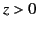.

4. A plane electromagnetic wave, linearly polarized in the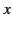-direction, and propagating in the-direction through an electrical conducting medium of conductivityand relative dielectric constant unity, is governed by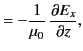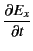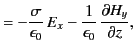where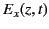and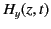are the electric and magnetic components of the wave. Derive an energy conservation equation of the form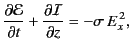where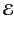is the electromagnetic energy per unit volume, and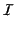the electromagnetic energy flux. Give expressions forand. What does the right-hand side of the previous equation represent? Demonstrate that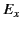obeys the wave-diffusion equationwhere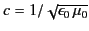. Consider the high frequency, low conductivity, limit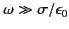. Show that a wave propagating into the medium varies as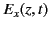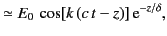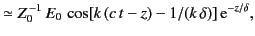where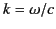,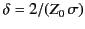, and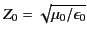. Demonstrate that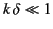: that is, the wave penetrates many wavelengths into the medium.

5. Consider a uniform plasma of plasma frequency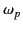containing a uniform magnetic field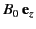. Show that left-hand circularly polarized electromagnetic waves can only propagate parallel to the magnetic field provided that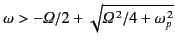, where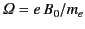is the electron cyclotron frequency. Demonstrate that right-hand circularly polarized electromagnetic waves can only propagate parallel to the magnetic field provided that their frequencies do not lie in the range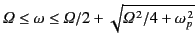.Next: Wave Propagation in Inhomogeneous Up: Wave Propagation in Uniform Previous: Signal Arrival
Richard Fitzpatrick 2014-06-27